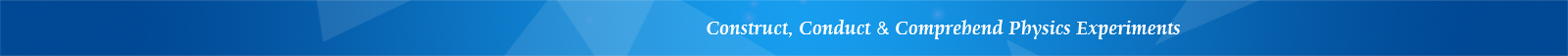## LEOI-32 Experimental System for Crystal Magneto-Optic Effect

Note: oscilloscope not included

Features

• Easy operation

• Precise measurement

• Stable base

• Magneto-optic modulation technique demo

Introduction

The magneto-optic rotation effect of a crystal, also called the Faraday effect, describes the effect of polarization rotation of an optical beam going through a layer of magneto-optic material. It plays an important role in optical experiments. This experimental system is designed to

1. Observe the magneto-optic rotation effect of a material under test

2. Understand the flow direction of a magnetic current vs the polarization rotation direction of a Faraday rotator

3. Calculate the Verdet constant

4. Demonstrate optical communication using magneto-optic modulation technique

This experimental system can be used to conduct the following experiments:

2. Calculate the Verdet constant of a material
3. Characterize a magneto-optic glass
4. Demonstrate optical communication using magneto-optic modulation technique